MOAMBE 2004 volume 169  number 2  Mathematics Member of the Academy L. Zhizhiashvili On Cauchy and Hilbert Singular Operators Presented September 16, 2003 ABSTRACT. In the paper some approximate properties of Cauchy singular operator for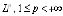, spaces are established. © 2004 Bull. Georg. Acad. Sci. Key words: Cauchy and Hilbert operators. Mathematics L. Alexidze, Z. Zerakidze On Some Properties of Strongly and Weakly Separable Statistical Structures Connected with a Stochastic System Presented by Corr. Member of the Academy E. Nadaraya, November 18, 2003 ABSTRACT. In the present paper we prove the necessary and sufficient conditions when statistical structures connected with a stochastic system are strongly and weakly separable. © 2004 Bull. Georg. Acad. Sci. Key words: Hilbert space of measures, weakly and strongly separable statistical structures. Mathematics V. Meskhia ON ABSOLUTELY CONVERGENT FOURIER SERIES WITH RESPECT TO HAAR MULTIPLE SEPARABLE WAVELET Presented by Member of the Academy L. Zhizhiashvili, July 8, 2003 ABSTRACT. In the given work we have established the necessary and sufficient condition on a function g, for the absolute convergence of the Haar multiple separable wavelet expansion of composition gBf for all f with absolutely convergent Haar multiple wavelet expansion. © 2004 Bull. Georg. Acad. Sci. Key words: multiple separable wavelet, multiresolution analysis, composition, absolute convergence. Mathematics D. Gubelidze On a Second Order Degenerate Elliptic System of Equations in Domain Containing an Angle Presented by Corr. Member of the Academy R.Bantsuri, Yuly 8, 2003 ABSTRACT. The paper deals with a system of second order elliptic differential equations which undergoes degeneration on the boundary of the domain. Existence and uniqueness of generalized solutions of different homogenous boundary problems in weighted Sobolev spaces are shown. © 2004 Bull. Georg. Acad. Sci. Key words: elliptic system with order degeneration, weighted Sobolev space. Mathematics L. Tibua
 AN ALGORITHM OF THE CLASSIFICATION OF PROPOSITIIONAL LOGIC FORMULAS Presented by Member of the Academy V. Chavchanidze, July 22, 2003 ABSTRACT. Formulas of propositional logic are proved as algorithmic processes. It is established that all these processes can be completed and their final values are one of the following halting constants: T (a valid formula), F (an inconsistent formula) and S (an indefinite formula). © 2004 Bull. Georg. Acad. Sci. Key words: Algorithmic process, disjunctive type, disjunctive part, conjunctive type, conjunctive part, algorithmic process by T-strategy, algorithmic process by F-strategy, algorithmic process by T+F-strategy, halting constant. Mathematics G. Giorgadze Euler characteristics of certain moduli spaces Presented by Member of the Academy G.Kharatishvili, October 20, 2003 ABSTRACT. We present an approach to the algorithmic computation of the Euler characteristic of configuration spaces of spatial polygons based on the signature formulae for the topological degree. Relationship is outlined between those configuration spaces and the moduli space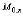of stable curves of genus 0 with marked points and its Deligne-Mumford compactification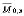. © 2004 Bull. Georg. Acad. Sci. Key words: Configuration space of polygon, linkage, Euler characteristic, topological degree, moduli space, signature formulae. Mathematics G. Giorgadze Euler characteristics of certain moduli spaces Presented by Member of the Academy G.Kharatishvili, October 20, 2003 ABSTRACT. We present an approach to the algorithmic computation of the Euler characteristic of configuration spaces of spatial polygons based on the signature formulae for the topological degree. Relationship is outlined between those configuration spaces and the moduli spaceof stable curves of genus 0 with marked points and its Deligne-Mumford compactification. © 2004 Bull. Georg. Acad. Sci. Key words: Configuration space of polygon, linkage, Euler characteristic, topological degree, moduli space, signature formulae. Mathematics I. Bakhia Dimensional Properties of Generalized Manifolds Presented by Member of the Academy N. Berikashvili, December 10, 2003 ABSTRACT. We consider dimensional type functions defined on the class of p-bicompact spaces. Using these functions, the dimensional properties of generalized manifolds are studied. The class of weakly net-like spaces is introduced, which is a natural generalization of net-like spaces in the sense of V. Proizvolov and the behavior of this class is studied in the case of (monotone, open) mappings of special type. Generalizations of V. Proizvolov's theorems are obtained for weakly net-like spaces. © 2004 Bull. Georg. Acad. Sci. Key words: dimensionality, manifold, Brauer's theorem, net-like space, weakly net-like space. Mathematics
 G. Tkebuchava Subsequences of Partial Sums of Multiple Fourier and Fourier-Walsh Series Presented by Member of the Academy L.Zhizhiashvili, September 9, 2003 ABSTRACT. The convergence in norm and in measure of subsequences of partial sums of multiple Fourier and Fourier-Walsh series is considered. © 2004 Bull. Georg. Acad. Sci. Key words: multiple Fourier series, Walsh system, convergence in measure, Orlicz space. Mathematics N. Chelidze The Banach-Steinhaus theorem for the double Denjoy integral Presented by Member of the Academy L. Zhizhiashvili,  September 16, 2003 Abstract. One of the theorems of the Banach-Steinhaus type to D-integrable function f of two variables is extended. © 2004 Bull. Georg. Acad. Sci. Key words: D-integrable function, ACG-function, a function of bounded variation. Mathematics A. Aplakov On the Absolute Convergence of the Multiple Series of Fourier-Haar Coefficients Presented by Member of the Academy L. Zhizhiashvili, June 24, 2003 ABSTRACT. In this paper we study an absolute convergence of the series of Fourier-Haar coefficients in the term of partial modulus of variation of function. Key words: Fourier-Haar coefficients, absolute convergence, multiple series, partial modulus of variation. Mathematics Sh. Bakhtadze On Generalized Projective Homologies of Locally Compact Paracompact Spaces Presented by Corr. Member D. Baladze, February 3, 2004 ABSTRACT. The projective homology theory is considered on the category of pairs of locally compact paracompact spaces and their proper mappings. In particular, groups of projective homologies with coefficients in an arbitrary pair of generalized topological groups are constructed. It is proved that the theory Hch satisfies the Steenrod-Eilenberg axioms. © 2004 Bull. Georg. Acad. Sci. Key words: projective homology, paracompact space. Mathematics Ya. Diasamidze, Sh. Makharadze, G. Partenadze, O. Givradze On Final X-Semilattices of Unions Presented by Member of the Academy D. Baladze, December 1, 2003
 Abstract. It is known that any commutative semigroup of idempotents is isomorphic to some Xsemilattice of union and X-semilattices of unions play important role while studying many abstract properties of complete semigroups of binary relations. In the work final X-semilattices of unions are studied by means of characteristic family of sets and characteristic mapping of X-semilattices of unions. © 2004 Bull. Georg. Acad. Sci. Key words: complete semigroups, binary relations, complete semilattices. Mathematics Ya. Diasamidze, Sh. Makharadze, G. Partenadze, O. Givradze On Final X-Semilattices of Unions Presented by Member of the Academy D. Baladze, December 1, 2003 Abstract. It is known that any commutative semigroup of idempotents is isomorphic to some Xsemilattice of union and X-semilattices of unions play important role while studying many abstract properties of complete semigroups of binary relations. In the work final X-semilattices of unions are studied by means of characteristic family of sets and characteristic mapping of X-semilattices of unions. © 2004 Bull. Georg. Acad. Sci. Key words: complete semigroups, binary relations, complete semilattices. Theory of elasticity A. Papukashvili Antiplane Problems of Theory of Elasticity for Piecewise-Homogeneous Orthotropic Plane Slackened with Cracks Presented by Corr. Member of the Academy R.Bantsuri, July 29, 2003 ABSTRACT. Antiplane problems of the theory of elasticity by using the theory of analytical functions are presented in the paper. These problems lead to a system of singular equations with immovable singularity with the respected to leap of the tangent stress. The problems of behavior of solutions at the boundary are studied. © 2004 Bull. Georg. Acad. Sci. Key words: cracks, peculiarity, singular equations, Cauchy type integral. Cybernetics D. Bashaleishvili Identification of Static Systems represented by Volterra Polynomials with Nonseparable Kernels Presented by Member of the Academy V. Chavchanidze, September 15, 2003 ABSTRACT. The solution of the problem of "black box" identification consists in searching for a mathematical model of the concrete diminishing (DS) or enlarging system (ES) in various classes of static systems (quasilinear systems, systems represented by Volterra polynomials with separable kernels and by those with nonseparable kernels). Methods of finding the desired mathematical model in the first two classes are developed by the author earlier. This paper deals with a method of finding the desired model in the class of Volterra polynomials with nonseparable kernels. © 2004 Bull. Georg. Acad. Sci. Key words: DS identification, ES identification, system of functional equations, separable kernel, nonseparable kernel, Volterra polynomial, operator restriction, projective method. Computer Science I. Javakhishvili, A. Kvitashvili
 Physics I. Titvinidze, Corr. Member of the Academy G. Japaridze CORRELATION FUNCTIONS OF THE SPIN S=1/2 EXTENDED XY MODEL Presented November 11, 2003 ABSTRACT. The large-distance asymptotic behavior of the spin-spin correlation functions of the spin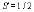extended XY model has been studied using the bosonization approach. The model describes the spin system on a zigzag chain, which is characterized in addition to the standard transverse exchange between spins on neighboring sites (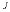), by the three-spin interaction. This three-spin coupling describes the "correlated exchange" interaction (), where the transverse exchange between spins on next-to-nearest neighbor sites depends on the "z" projection of an intermediate spin. The quantum phase transition, separating two different spin-liquid phases, takes place in the ground state of the system at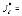2J. In this paper we study in detail the large-distance behavior of various spin-spin correlation functions in these two spin-liquid phases. © 2004 Bull. Georg. Acad. Sci. Kew words: spin systems, quantum phase transitions. Geophysics L. Javakhishvili Comparison of the Anomalies of ra Fields Above the Conductive Sphere for Different Separation of the D.C. Device Operating in Non-Stable Regime of ra in the Range of Critical Point Presented by Corr. Member of the Academy T. Chelidze, June 24, 2003 ABSTRACT. Every separation of the device operating in non-stable regime of ra is characterized by the critical value of the parameter sc. For the given separation of the device by choosing the parameter sc the characteristic parameters of the device approximate to the parameter of the immersed sphere, due to which the result dependes on the information of the existence of sphere. © 2004 Bull. Georg. Acad. Sci. Key words: non-stable regime of ra, critical point, direct current, electric prospecting, apparent resistivity, specific resistivity. Geophysics I. Shekriladze Thermo-Hydrodynamical Alignment Effect, Conditions of Realization Presented by Member of the Academy B. Balavadze, January 22, 2004 ABSTRACT. Conditions are considered of rapid intensification of a tropical hurricane. Characteristic range is determined of equilibrium translation speed. Dependence is considered of intensity of a tropical hurricane from alignment number and hurricane heat potential. The basic assumption is confirmed of developed previously equilibrium translation model. © 2004 Bull. Georg. Acad. Sci. Key words: tropical hurricane, alignment effect, equilibrium translation, alignment number. Organic Chemistry T. Khuchua, T. Doksopulo, E. Chikvaidze, Corr. Member of the Academy Sh. Samsoniya Unsaturated hydrocarbonS receiving from